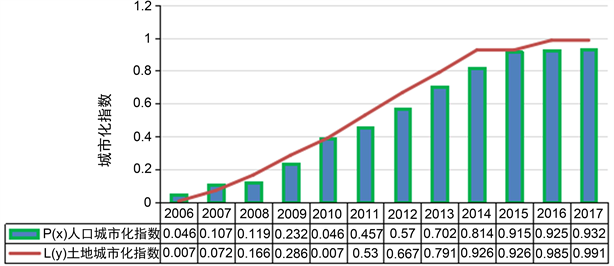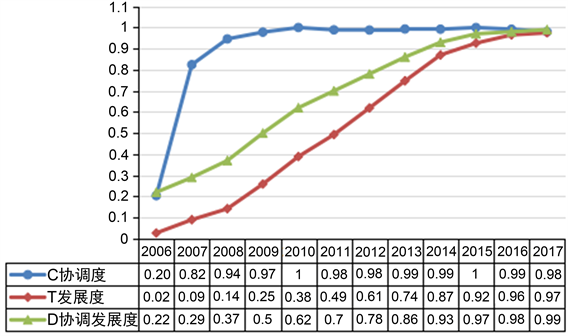﻿ 西部生态脆弱地区人口城市化与土地城市化协调水平研究——以云南省为例

# 西部生态脆弱地区人口城市化与土地城市化协调水平研究——以云南省为例An Analysis on Coordinated Development between Population Urbanization and Land Urbanization in Yunnan Province

Abstract: The coordinated development of population urbanization and land urbanization has become an important research area. In this paper, an evaluation index system is constructed in order to calculate the 2006 to 2017 population urbanization index and land urbanization index in Guangdong Province. The results show that the population urbanization and land urbanization in Yunnan Province are at a low level, but the overall trend is on the rise. The difference between population and land urbanization index is gradually narrowing. The level of coordinated development shows an increasing trend. In view of this situation, this paper puts forward relevant countermeasures and suggestions for the coordinated development of population urbanization and land urbanization in Yunnan Province.

1. 引言

2. 研究方法

2.1. 指标体系

Table 1. The index system of population urbanization and land urbanization

2.2. 数据来源

${{X}^{\prime }}_{ij}=\left\{\begin{array}{l}\left({X}_{ij}-{m}_{i}\right)/\left({M}_{i}-{m}_{i}\right)\text{\hspace{0.17em}}\text{\hspace{0.17em}}\text{\hspace{0.17em}}\text{\hspace{0.17em}}\text{\hspace{0.17em}}\text{\hspace{0.17em}}\text{\hspace{0.17em}}\left(正向指标\right)\\ \left({M}_{i}-{X}_{ij}\right)/\left({M}_{i}-{m}_{i}\right)\text{\hspace{0.17em}}\text{\hspace{0.17em}}\text{\hspace{0.17em}}\text{\hspace{0.17em}}\text{\hspace{0.17em}}\text{\hspace{0.17em}}\left(负向指标\right)\end{array}$ (1)

2.3. 主成分分析及权重确定

${a}_{i}=\frac{|{A}_{i}|}{\underset{i=1}{\overset{n}{\sum }}|{A}_{i}|}$${b}_{j}=\frac{|{B}_{j}|}{\underset{j=1}{\overset{m}{\sum }}|{B}_{j}|}$ (2)

$P\left(x\right)=\underset{i=1}{\sum }{a}_{i}{x}_{i}$$L\left(y\right)=\underset{j=1}{\sum }{b}_{j}{y}_{j}$ (3)Table 2. Principal component variance load2.4. 协调度指数的计算

$C={\left\{\frac{P\left(x\right)\ast L\left(y\right)}{{\left[\frac{P\left(x\right)+L\left(y\right)}{2}\right]}^{2}}\right\}}^{k}$ (4)

$T=\alpha P\left(x\right)+\beta L\left(y\right)$ (5)

$D=\sqrt{C\ast T}$ (6)

2.5. 协调类型的判定Table 4. Coordinate type classification criteria

3. 云南省人口城市化与土地城市化协调发展现状评价Table 5. Evaluation results of coordinated development of urbanization in Yunnan provinceFigure 1. Population urbanization and land city index change in Yunnan province from 2006 to 2017Figure 2. Change curve of urbanization coordination development degree in Yunnan from 2006 to 2017

1) 2006~2008年云南省人口与土地城市化发展属于失调类型。人口城市化水平比土地城市化水平发展慢，但该阶段云南人口城市化规模大于土地城市化规模，因此属于土地城市化质量滞后型。2006~2008年云南人口城市化发展度与土地城市化发展度T均低于协调度C，整体协调发展度水平较低，因此判定2006~2008年期间云南人口城市化与土地城市化属于失调发展。

2) 2009年云南省人口与土地城市化发展属于过渡类型。2009年云南人口城市化与土地城市化协调度指数0.978，发展度指数0.259，发展度低于协调度，协调发展度D为0.5，仍处于较低水平，根据协调类型划分标准，该阶段处于过渡阶段；与2006~2008年相比，本阶段云南人口城市化与土地城市化指数都有增长，且土地城市化指数大于人口城市化指数，但整体仍处于较低发展水平，尤其是这一阶段人口城市化发展程度较低，属于人口城市化滞后型。

3) 2010~2017年云南人口与土地城市化发展属于协调型。本阶段云南人口城市化与土地城市化协调度C相对稳定，波动范围小且最大值为1，发展度T从2010年的0.389增长到2015年0.975，发展度增长快，协调发展度D从2010年的0.62 (初级协调)增长到2017年的0.99 (优质协调)，此结果表明，2010~2017年期间，云南人口城市化与土地城市化协调发展程度稳步提升且协调发展趋势越来越明显。另外，图1可以看出该阶段土地城市化指数大于人口城市化指数，此阶段云南省土地城市化类型属于土地城市化超前发展型。

4. 总结与讨论

1) 整体来看，云南省人口城市化和土地城市化水平低，政府应推动加快云南省城市化进程。合理控制昆明城市规模，重点推动滇中城市群一体化发展。积极促进怒江、迪庆、西双版纳等边疆民族地区的城市化水平，这也符合中国城市发展的总方针要求。

2) 依据协调发展现状，云南省应合理控制土地城市化的进程，适当减慢城市建设用地的增长速度，加快提升土地利用效率。首先，合理规划城市用地规模，坚持可持续发展，加大限制郊区乡村土地向城镇土地的转变。其次，加快清理土地权属与使用状况，对空闲的城市建设用地及时进行检查与整改。依靠市场的作用，将土地利用方式向规模经营转变。

3) 针对云南省当前人口城市化滞后的问题，在今后城市发展中应坚持以人为本，加快户籍制度的改革，打破城乡二元结构弊端，重点解决城市化中居民的社会生活保障问题，促进人口城市化水平的提升。其次，纠正城市化的传统理念，以人口城市化为核心，努力实现城乡管理一体化，缩小城乡发展差距。

NOTES

*通讯作者。

 淡永利. 湖北省人口城市化与土地城市化协调发展研究[D]: [硕士学位论文]. 武汉: 华中师范大学, 2014.

 邓琛, 董利民, 等. 咸宁市人口城市化与土地城市化协调发展评价[J]. 湖北农业科学, 2015(15): 3810-3815.

 苑韶峰, 杨丽霞, 王雪禅. 人口城镇化与土地城镇化协调发展的空间差异研究——以浙江省69县市为例[J]. 中国土地科学, 2013(11): 18-22.

Top